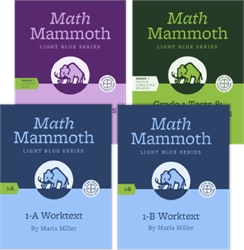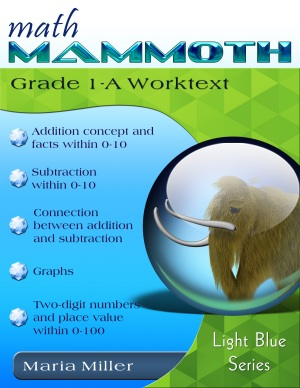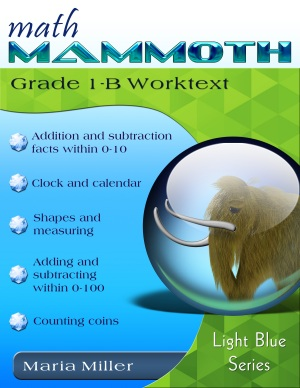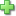Math Mammoth 1 - Bundle

by Maria Miller
Publisher: Math Mammoth
Item: 67805
Current Retail Price: \$65.75
Not in stock

See series description for full review.

Math Mammoth Grade 1 Complete Curriculum is a complete math program for 1st grade. It consists of two student worktexts (A and B), a separate answer key for each, tests, cumulative reviews, and in the download version, an additional worksheet maker (Internet access required) to make extra practice worksheets when needed.145 pages
129 lesson pages,
(of which 8 are an
optional Kindergarten review)144 pages
125 lesson pages

1-A contents and samples
1-B contents and samples

The four main areas of study for first grade are:

1. The concepts of addition and subtraction, and strategies for addition and subtraction facts (chapters 1-2; chapter 4);
2. Developing understanding of whole number relationships andplace value till 100 (chapter 3 and chapter 7);
3. Developing understanding of measuring lengths as iterating length units (chapter 6); and
4. Reasoning about attributes of geometric shapes, such as the number of sides and the number of corners, and composing and decomposing geometric shapes (chapter 6).

Additional topics we study in first grade are clock to the half hour (chapter 5) and counting coins (chapter 8).

Please also see the table of contents for 1-A and 1-B (in the sample files), which will let you see the topics covered in more detail.

Some main features of Math Mammoth complete curriculum (Light Blue series) are:

• focuses on understanding of mathematical concepts
• uses clear explanations, lots of visual exercises and pattern exercises
• mastery oriented: concentrates fairly long on a topic, with fairly few topics per grade
• emphasizes mental math and developing number sense
• very little teacher preparation needed

Manipulatives

Here is a list of manipulatives that are needed or recommended for grade 1:

1. 100-bead abacus (for 1-A and 1-B; especially the chapter on place value and chapter 7)Click here to write a review